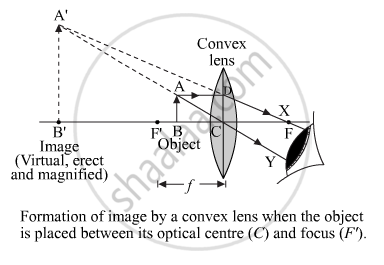Share

# Draw Ray Diagrams to Represent the Nature, Position and Relative Size of the Image Formed by a Convex Lens for the Object Placed: Between F1 And the Optical Centre O of the Lens. Which of the Above Two Cases Shows the Use of Convex Lens as a Magnifying Glass? Give Reasons for Your Choice. - CBSE Class 10 - Science

ConceptRepresentation of Images Formed by Spherical Mirrors Using Ray Diagrams - Convex Mirror

#### Question

Draw ray diagrams to represent the nature, position and relative size of the image formed by a convex lens for the object placed:

between F1 and the optical centre O of the lens.
Which of the above two cases shows the use of convex lens as a magnifying glass? Give reasons for your choice.

#### Solution

When the object is placed between F1 and the optical centre O of the lens, the image formed by the convex lens is behind the object (left of the lens). This image is virtual, magnified and erect as shown in the figure.is used as a magnifying glass. The reason being, the image of an object that is placed between F1 and the optical centre is virtual and magnified, which is the requirement of a magnifying glass.

Is there an error in this question or solution?

#### APPEARS IN

Solution Draw Ray Diagrams to Represent the Nature, Position and Relative Size of the Image Formed by a Convex Lens for the Object Placed: Between F1 And the Optical Centre O of the Lens. Which of the Above Two Cases Shows the Use of Convex Lens as a Magnifying Glass? Give Reasons for Your Choice. Concept: Representation of Images Formed by Spherical Mirrors Using Ray Diagrams - Convex Mirror.
S